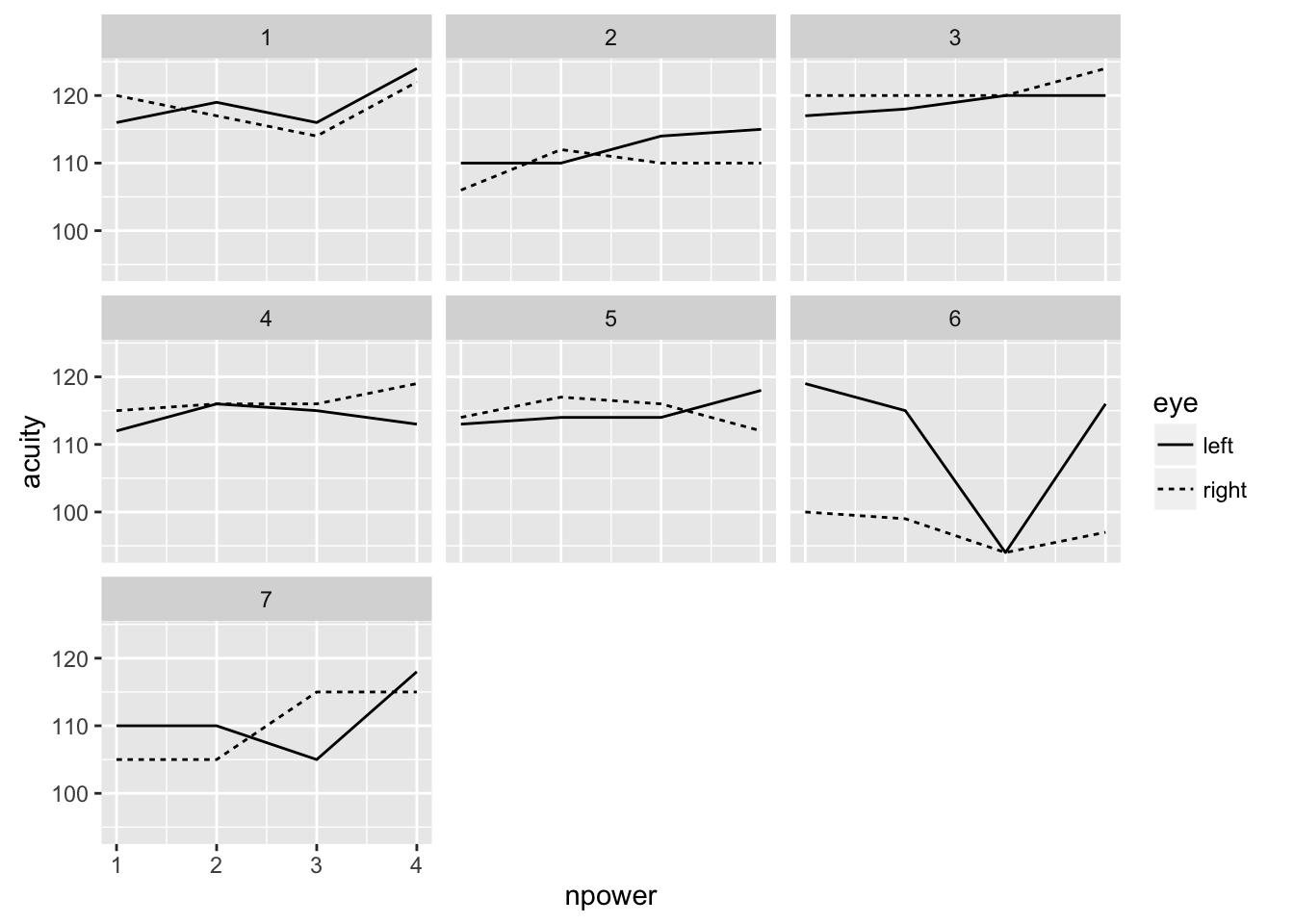See the introduction for an overview. Also see other analyses of this data. See the textbook for a full discussion.

``````library(ggplot2)
library(rstan)
library(reshape2)
library(dplyr)``````

# Data

Load in and summarize the data:

``````data(vision, package="faraway")
vision\$npower <- rep(1:4,14)
summary(vision)``````
``````     acuity     power       eye     subject     npower
Min.   : 94   6/6 :14   left :28   1:8     Min.   :1.00
1st Qu.:110   6/18:14   right:28   2:8     1st Qu.:1.75
Median :115   6/36:14              3:8     Median :2.50
Mean   :113   6/60:14              4:8     Mean   :2.50
3rd Qu.:118                        5:8     3rd Qu.:3.25
Max.   :124                        6:8     Max.   :4.00
7:8                   ``````

Plot the data:

``ggplot(vision, aes(y=acuity, x=npower, linetype=eye))+geom_line()+facet_wrap(~ subject)``# Fitting

Fit the model. Requires use of STAN command file multilevel.stan. We have used uninformative priors for the treatment effects but slightly informative half-cauchy priors for the three variances. The fixed effects have been collected into a single design matrix. We are using the same STAN command file as for the multilevel.html multilevel example because we have the same two levels of nesting.

``````lmod <- lm(acuity ~ power, vision)
sdscal <- sd(residuals(lmod))
Xmatrix <- model.matrix(lmod)
vision\$subjeye <- factor(paste(vision\$subject,vision\$eye,sep="."))
visdat <- list(Nobs=nrow(vision),
Npreds=ncol(Xmatrix),
Nlev1=length(unique(vision\$subject)),
Nlev2=length(unique(vision\$subjeye)),
y=vision\$acuity,
x=Xmatrix,
levind1=as.numeric(vision\$subject),
levind2=as.numeric(vision\$subjeye),
sdscal=sdscal)``````

Break the fitting of the model into three steps.

``````rstan_options(auto_write = TRUE)
options(mc.cores = parallel::detectCores())
rt <- stanc("multilevel.stan")
sm <- stan_model(stanc_ret = rt, verbose=FALSE)
set.seed(123)
system.time(fit <- sampling(sm, data=visdat))``````
``````   user  system elapsed
0.351   0.088   8.101 ``````

# Diagnostics

For the error SD:

``````pname <- "sigmaeps"
muc <- rstan::extract(fit, pars=pname,  permuted=FALSE, inc_warmup=FALSE)
mdf <- melt(muc)
ggplot(mdf,aes(x=iterations,y=value,color=chains)) + geom_line() + ylab(mdf\$parameters)``````